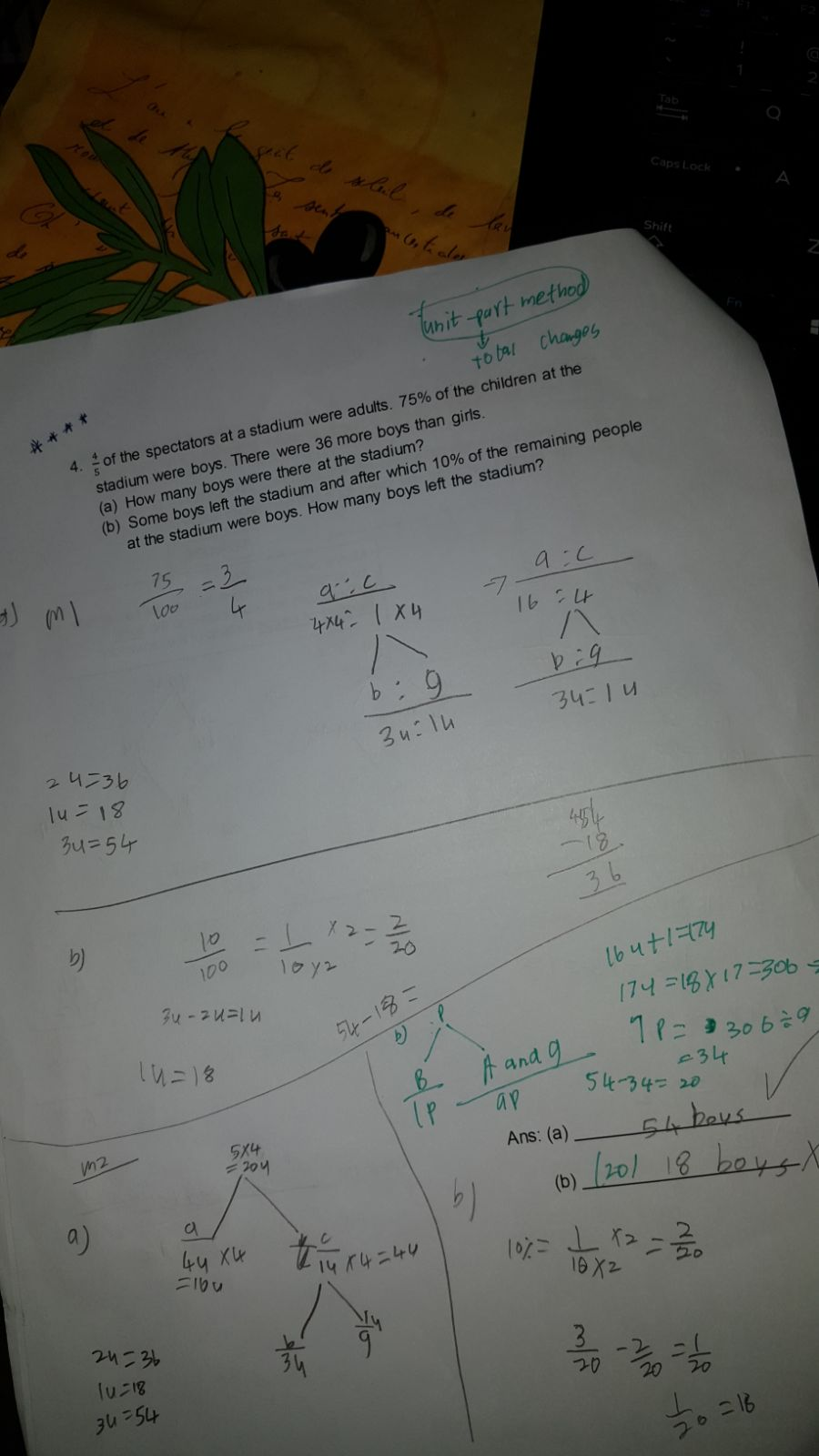# Question

4/5 of the spectators at a stadium were adults. 75% of the children at the stadium were boys. There were 36 more boys than girls.

(a) How many boys were there at the stadium ?   (b) Some boys left the stadium and after which 10% of the remaining people at the stadium were boys. How many boys left the stadium ?

A  :  C  :  Total             B  :  G  :  Total C

4  :  1  :  5                     3  :  1  :  4

×4 |  :  |×4

16  :  4

3u – 1u = 2u

2u = 36

1u = 36÷2

= 18

a) number of boys = 3u

= 3×18

= 54

Total A + C = 20u

= 360

A +G  :  B  :  Total

16+1  :  3

17   :   3

×9    153 :    27   180

|     -?|

9     :    1

×17  153  :   17

180u = 360

1u = 2

b) Boys left = 27u – 17u

= 10u

= 10 × 2

= 20 ( ans)

1 Reply 1 Like

My DS has written different answer for question b. can you pl. check.1 Reply 0 Likes